# Estimate Model Parameters Per Experiment (GUI)

This example shows how to use multiple experiments to estimate a mix of model parameter values; some that are estimated using all the experiments and others that are estimated using individual experiments. The example also shows how to configure estimation experiments with experiment dependent parameter values.

You estimate the parameters of a rechargeable battery based on data collected in experiments that discharge and charge the battery.

### Open the Model and Get Experimental Data

This example estimates parameters of a simple, rechargeable battery model, `sdoBattery`. The model input is the battery current and the model output, the battery terminal voltage, is computed from the battery state-of-charge.

`open_system('sdoBattery')`The model is based on the equation

`$\mathit{E}=\left(1-\mathrm{Loss}\right)\mathit{V}-\mathit{K}{\mathit{Q}}_{\mathrm{max}}\frac{1-\mathit{s}}{\mathit{s}}$`

In the equation:

$\mathit{E}$ is the battery terminal voltage in Volts.

$\mathit{V}$ is the battery constant voltage in Volts.

$\mathit{K}$ is the battery polarization resistance in Ohms.

${\mathit{Q}}_{\mathrm{max}}$ is the maximum battery capacity in Ampere-hours.

$\mathit{s}$ is the battery charge state, with 1 being fully charged and 0 discharged. The battery state-of-charge is computed from the integral of the battery current with a positive current indicating discharge and a negative current indicating charging. The battery initial state-of-charge is specified by ${\mathit{Q}}_{0}$ in Ampere-hours.

$\mathrm{Loss}$ is the voltage drop when charging, expressed as a fraction of the battery constant voltage. When the battery is discharging this value is zero.

`V`, `K`, `Qmax`, `Q0`, and `Loss` are variables defined in the model workspace.

### Estimation Experiment Data

A 1.2V (6500mAh) battery was subjected to a discharge and a charging experiment. This experiment data has been loaded into a preconfigured estimation session.

Use the following commands to load the pre-configured estimation session.

```load sdoBattery_spesession spetool(SDOSessionData) ```

The measured charge and discharge experiment data are loaded and plotted. Click the View tab to layout the plots so that the `Experiment plot:Charge_Exp` and `Experiment plot:DCharge_Exp` are both visible. Click Plot Model Response to see how well the model simulation matches the measured experiment data.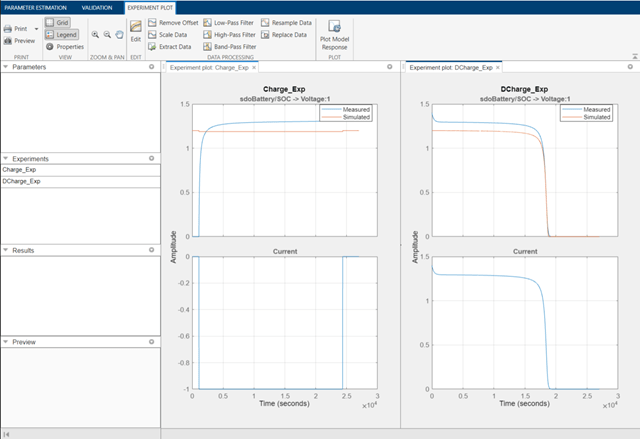The plots show that the battery initial charge `Q0` is not set correctly for the `Charge_Exp` experiment and that the model `V`, `K`, and `Loss` parameters need to be estimated.

### Setting Experiment Parameter Values

The previous plot indicates that the `Charge_Exp` battery initial charge, `Q0`, is not set correctly. Add the initial charge to both experiments. Right click `Charge_Exp` and select Edit. A dialog to edit the experiment opens.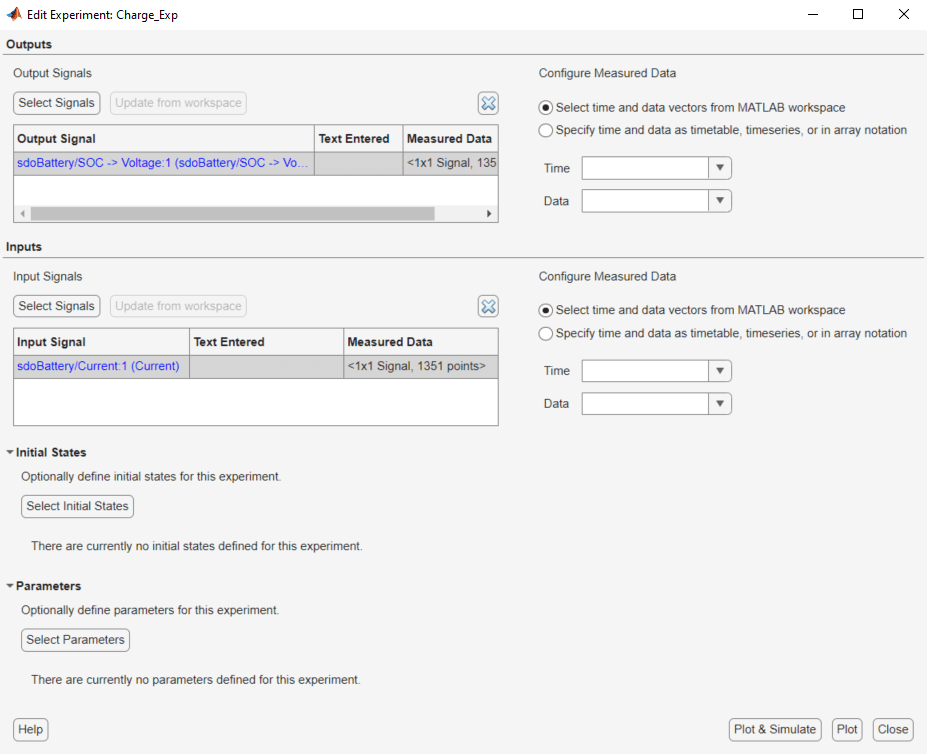Click Select Parameters to open a dialog to add model parameters to the experiment. Select `Loss` and `Q0` to add to the experiment. Select `Loss` as we need to estimate this parameter using only the `Charge_Exp` experiment. Click Ok to add the `Q0` and `Loss` parameters to the experiment.Set the battery initial charge `Q0` in the `Charge_Exp` to 0, i.e. there is no initial charge.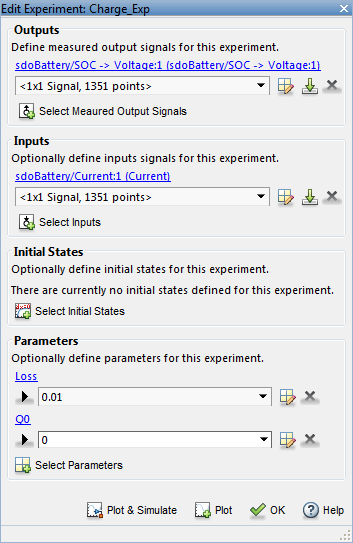Similarly add the battery initial charge `Q0` to the `DCharge_Exp` experiment and set the initial charge to 6.5., i.e. for this experiment there is an initial charge.Now that the experiments are updated with the correct initial battery charge click Plot Model Response to simulate the model and compare measured and simulated data.The experiment plots show that the experiment initial conditions match but the battery response does not. The next step is to estimate the `K` and `V` model parameters.

### Select Estimation Parameters

The previous plot showed that the model response does not match the measured data and we need to estimate the model `V` and `K` parameters.

Click Select Parameters to open a dialog to select model parameters.The upper portion of the select parameters dialog has a section for parameters that are tuned using all experiments. Click Select Parameters and add the `V` and `K` model parameters to the estimated parameters. Set the `V` minimum to 0 and the maximum to 2, similarly set the `K` minimum to 1e-6 and maximum to 0.1.The lower section of the dialog has a section for initial states and parameters that are tuned using individual experiments.

For the `Charge_Exp` we tune the `Loss` parameter and set its minimum to 0 maximum to 0.5. The battery initial charge `Q0` is fixed to 0 and should not be estimated; uncheck Estimate.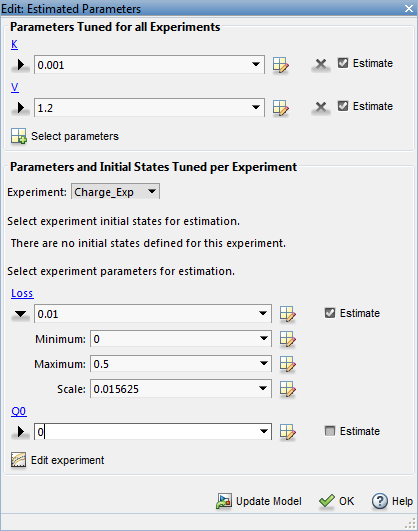Select `DCharge_Exp` from the Experiment combobox to view the parameter settings for the `DCharge_Exp` experiment. The battery initial charge `Q0` is fixed to 6.5 and should not be estimated; uncheck Estimate.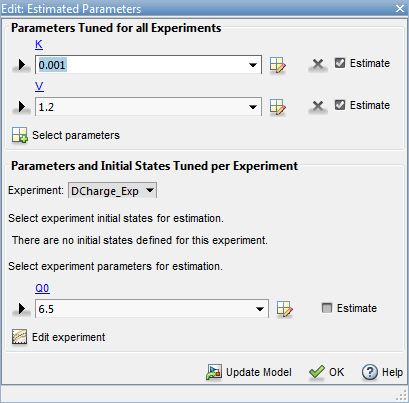### Estimate Parameter Values

The experiments and estimated parameters are configured and we a ready to run the estimation. First create a plot to monitor the estimation progress. Click Add Plot and select Parameter Trajectory. This creates a plot that shows how the estimated parameter values change during estimation. Click the View tab to layout the plots so that the experiment and parameter trajectory plots are all visible.Click the Estimate button to start the estimation. You can modify estimation options by setting the Cost Function combobox and clicking More Options.

While the estimation is running the plots update and a dialog showing estimation progress appears. The progress dialog shows the estimation iterations, the number of times the model has been evaluated (F-count), and the estimation cost at each iteration.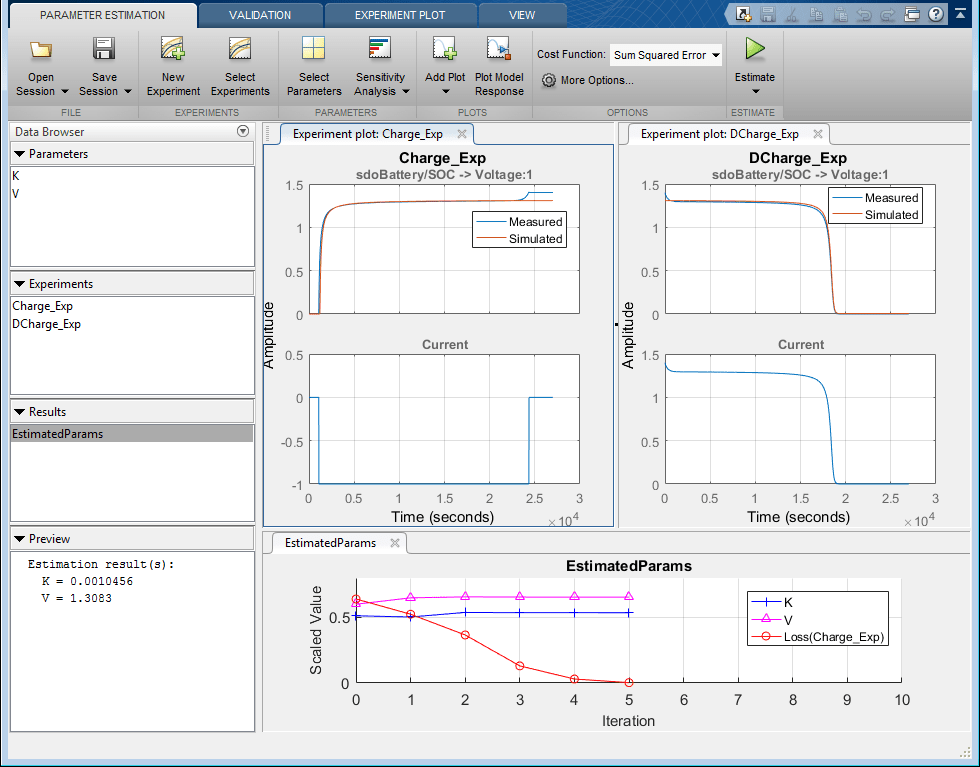After a number of iterations the estimation converges and terminates. The experiment plots show the measured and simulation data matching well. The `EstimatedParams` plot shows the `V`, `K`, and `Loss` parameters changing during the estimation; the scale of `V`, `K`, and `Loss` are different, right click on the plot and select Show scaled values to see how all the parameters changed from their original values.

### Related Examples

To learn how to estimate parameters per experiment using the `sdo.optimize` command, see Estimate Model Parameters Per Experiment (Code).

Close the model

`bdclose('sdoBattery')`